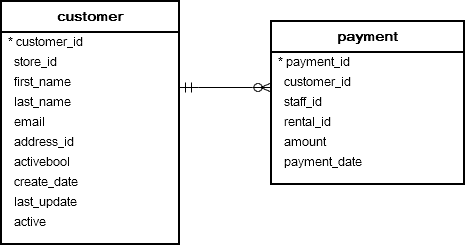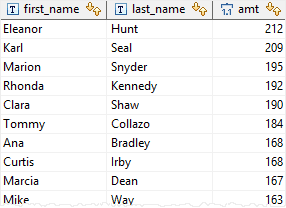# PostgreSQL Tutorial: CEIL Function

August 7, 2023

The PostgreSQL `CEIL()` function returns a number rounded up to the next whole number.

## Syntax

The following illustrates the syntax of the `CEIL()` function:

``````CEIL(numeric_expression)
``````

## Arguments

The `CEIL()` function requires one argument:

1) `numeric_expression`

The `numeric_expression` is a number (or an expression which evaluates to a number) that is rounded up.

## Return Value

The `CEIL()` function returns a value whose data type is the same as the input argument.

## Examples

The following statement illustrates how to use the `CEIL()` function to round a number up to the nearest integer:

``````SELECT
CEIL( 200.25 );
``````

The result is:

``````201
``````

Let’s take the `customer` and `payment` tables in the sample database for the demonstration.The following example calculates the ceiling of amounts paid by customers for rentals:

``````SELECT
first_name,
last_name,
CEIL(SUM( amount )) amt
FROM
payment
INNER JOIN customer
USING(customer_id)
GROUP BY
customer_id
ORDER BY
amt DESC;
``````

The following picture illustrates the result:## Remarks

To round a number down to the nearest whole number, you use the `FLOOR()` function.

In this tutorial, you have learned how to use the PostgreSQL `CEIL()` function to round a number up to the nearest integer, which is greater than or equal to the number.